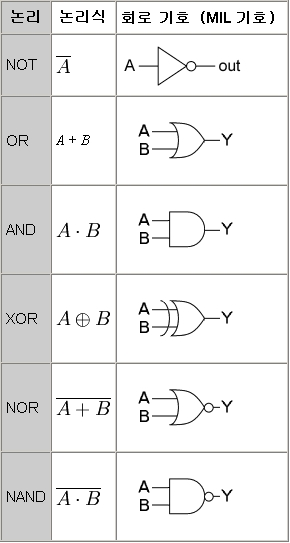# Logic Gate – 논리 회로

논리 회로는 불 대수(논리 연산)를 실시하는 회로 또는 디지탈 신호를 기억하는 회로 혹은 두가지 기능을 가지는 디지털 회로이다.

A logic gate takes one or more logic-level inputs and produces a single logic-level output. Because the output is also a logic level, an output of one logic gate can connect to the input of one or more other logic gates.

In electronic logic, a logic level is represented by a certain voltage (which depends on the type of electronic logic in use). Each logic gate requires power so that it can source and sink currents to achieve the correct output voltage. In logic circuit diagrams the power is not shown, but in a full electronic schematic, power connections are required. There are 7 positive logic gates and each gate has two laws or rules.논리 회로의 설계
논리 회로의 설계는 논리식이나 진리표가 사용된다.
좀더 회로도적인 표기 수단으로 MIL 기호 등 논리 소자
기호가 사용되었다.

1960년대에 등장한 표준 논리 IC (TI의 7400 시리즈)에
의하여 아날로그 회로 설계와 논리 설계를 분리하여
단순하게 구현이 가능하게 되었다.

작은 규모에서는 논리 소자 기호로 설계가 가능하지만,
큰 규모가되면 힘들어 진다. 그렇기 때문에 1990년대부터
대규모 회로 설계에는 하드웨어 기술 언어가 사용되고
있다. 그리고 집적 회로 기술의 발전에 대응할 수 있도록
다양한 모델에 적용이 가능한 독립적인 모델(동작 기술)을
사용하여 설계한다.

1990년대 후반에는 개발품의 경우 논리 회로 프로그램을 이용하여 PLD, CPLD, FPGA을 사용하였다. 양산품이나 고성능이 요구될 경우 ASIC를 사용하기도 했다.

Logic gates and hardware
NAND and NOR logic gates are the two pillars of logic, in that all other types of Boolean logic gates (i.e., AND, OR, NOT, XOR, XNOR) can be created from a suitable network of just NAND or just NOR gate(s). They can be built from relays or transistors, or any other technology that can create an inverter and a two-input AND or OR gate. Hence the NAND and NOR gates are called the universal gates.

DeMorgan equivalent symbols
By use of De Morgan’s theorem, an AND gate can be turned into an OR gate by inverting the sense of the logic at its inputs and outputs. This leads to a separate set of symbols with inverted inputs and the opposite core symbol. These symbols can make circuit diagrams for circuits using active low signals much clearer and help to show accidental connection of an active high output to an active low input or vice-versa.

Storage of bits
Related to the concept of logic gates (and also built from them) is the idea of storing a bit of information. The gates discussed up to here cannot store a value: when the inputs change, the outputs immediately react. It is possible to make a storage element either through a capacitor (which stores charge due to its physical properties) or by feedback. Connecting the output of a gate to the input causes it to be put through the logic again, and choosing the feedback correctly allows it to be preserved or modified through the use of other inputs. A set of gates arranged in this fashion is known as a “latch”, and more complicated designs that utilise clocks (signals that oscillate with a known period) and change only on the rising edge are called edge-triggered “flip-flops”. The combination of multiple flip-flops in parallel, to store a multiple-bit value, is known as a register. When using any of these gate setups the overall system has memory; it is then called a sequential system since its output can be influenced by its previous state(s).

These registers or capacitor-based circuits are known as computer memory. They vary in performance, based on factors of speed, complexity, and reliability of storage, and many different types of designs are used based on the application.

출처: 위키사전(logic gate), 위키사전(논리회로)